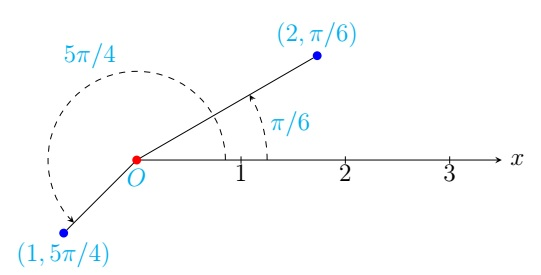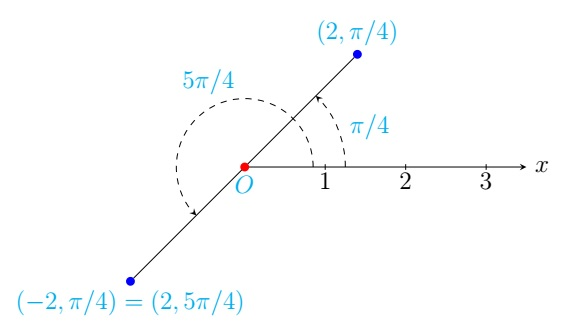Calculus II Home

## Polar Coordinates

We introduce a new coordinate system called the polar coordinate system which is useful in certain situations like dealing with circles or circular regions. The polar coordinate system has a fixed point called the pole (or the origin) denoted by $$O$$ and a fixed horizontal line from the pole called the polar axis (or the positive $$x$$-axis). The polar coordinates of a point $$P$$ in the plane are written as $$(r,\theta)$$ where $$r\geq 0$$ is the distance between $$P$$ and the pole (or the origin) and $$\theta$$ is the angle in $$[0,2\pi)$$ that is between the polar axis (or the positive $$x$$-axis) and the line joining $$P$$ and the pole (or the origin). Here an angle is positive when it is measured counterclockwise. Note that $$r=0$$ or $$(0,\theta)$$ correspond to the pole or the origin for any value of $$\theta$$.Note the following convention for polar coordinates not in the above unique representation of $$(r,\theta)$$:

1. $$(r,\theta)=(r,\theta+2n\pi)$$ for all integers $$n$$.

2. $$(-r,\theta)$$ is $$(r,\theta+\pi)$$ when $$0\leq \theta < \pi$$ and $$(r,\theta-\pi)$$ when $$\pi\leq \theta < 2\pi$$.

Example.

1. $$\left( 2,\frac{\pi}{4} \right)=\left( 2,\frac{9\pi}{4} \right)$$ as $$\frac{9\pi}{4}=2\pi+\frac{\pi}{4}$$. Similarly $$\left( 2,\frac{\pi}{4} \right)=\left( 2,-2\pi+\frac{\pi}{4} \right)=\left( 2,-\frac{7\pi}{4} \right)$$.

2. $$\left( -2,\frac{\pi}{4} \right)=\left( 2,\frac{\pi}{4}+\pi \right)=\left( 2,\frac{5\pi}{4}\right)$$.Conversion between Polar and Cartesian Coordinates:
The Cartesian coordinates $$(x,y)$$ of the polar coordinates $$(r,\theta)$$ are given by $x=r\cos \theta,\; y=r\sin \theta.$ Note from the preceding equations that $x^2+y^2=r^2\cos^2 \theta+r^2\sin^2 \theta=r^2(\cos^2 \theta+\sin^2\theta)=r^2$ and $\displaystyle\frac{y}{x}=\frac{r\sin \theta}{r\cos \theta}=\tan \theta.$ The polar coordinates $$(r,\theta)$$ of the Cartesian coordinates $$(x,y)$$ are give by $r=\sqrt{x^2+y^2},\; \theta=\tan^{-1}\left( \frac{y}{x} \right),$ where $$\theta$$ is the angle in $$[0,2\pi)$$ that is between the positive $$x$$-axis and the line joining $$(x,y)$$ and the origin. Note that $$-\frac{\pi}{2} <\tan^{-1}\left( \frac{y}{x} \right) < \frac{\pi}{2}$$ and there are two angles in $$[0,2\pi)$$ that satisfy $$\tan \theta=\displaystyle\frac{y}{x}$$. Therefore, when $$x < 0$$, the correct $$\theta$$ is $$\tan^{-1}\left( \frac{y}{x} \right) +\pi$$.

Example.

1. Find the Cartesian coordinates of the following points in polar coordinates: (a) $$(2,\pi/4)$$, (b) $$(4,-11\pi/6)$$.

2. Find the polar coordinates $$(r,\theta)$$ (with $$r\geq 0,\: 0\leq \theta < 2\pi$$) of the following points: (a) $$(\sqrt{3},1)$$, (b) $$(-2,2)$$.

Solution.

1. (a) Here $$r=2$$ and $$\theta=\frac{\pi}{4}$$. $x=2\cos\left( \frac{\pi}{4} \right)=2\frac{\sqrt{2}}{2}=\sqrt{2},\; y=2\sin \left( \frac{\pi}{4} \right)=2\frac{\sqrt{2}}{2}=\sqrt{2}.$ So the point $$(2,\pi/4)$$ in polar coordinates is $$(\sqrt{2},\sqrt{2})$$ in Cartesian coordinates.

(b) Here $$r=4$$ and $$\theta=-\frac{11\pi}{6}$$. \begin{align*} x &=4\cos\left( -\frac{11\pi}{6} \right)=4\cos\left( \frac{\pi}{6}-2\pi \right)=4\cos\left( \frac{\pi}{6} \right)=4 \frac{\sqrt{3}}{2}=2\sqrt{3},\\ y &=4\sin\left( -\frac{11\pi}{6} \right)=4\sin\left( \frac{\pi}{6}-2\pi \right)=4\sin\left( \frac{\pi}{6} \right)=4 \frac{1}{2}=2. \end{align*} So the point $$(4,-11\pi/6)$$ in polar coordinates is $$(2\sqrt{3},2)$$ in Cartesian coordinates.

2. (a) Here $$x=\sqrt{3}$$ and $$y=1$$. $r=\sqrt{(\sqrt{3})^2+1^2}=2,\; \theta=\tan^{-1}\left( \frac{1}{\sqrt{3}} \right)=\frac{\pi}{6}.$ So the point $$(\sqrt{3},1)$$ in Cartesian coordinates is $$(2,\frac{\pi}{6})$$ in polar coordinates.

(b) Here $$x=-2$$ and $$y=2$$. $r=\sqrt{(-2)^2+2^2}=2\sqrt{2},\; \theta=\tan^{-1}\left( \frac{2}{-2} \right)=\tan^{-1}(-1)=-\frac{\pi}{4}.$ Note that $$\theta=-\frac{\pi}{4}$$ is not correct as it corresponds to the fourth quadrant where the point $$(-2,2)$$ lies in the second quadrant. So the correct $$\theta$$ is $$\theta=-\frac{\pi}{4}+\pi=\frac{3\pi}{4}$$. Thus the point $$(-2,2)$$ in Cartesian coordinates is $$(2\sqrt{2},\frac{3\pi}{4})$$ in polar coordinates.

The Polar Equation of a Curve:
The equation of a curve in rectangular coordinates can be converted to a polar equation by substituting $$x=r\cos \theta,\; y=r\sin \theta$$. A polar equation is written in the form $$r=f(\theta)$$ or $$F(r,\theta)=0$$.

Example.

1. The polar equation of the circle $$x^2+y^2=a^2$$, $$a > 0$$ is $$r=a$$.
Since $$x^2+y^2=r^2$$, $$r^2=a^2 \implies r=a$$.

2. The polar equation of the circle $$(x-a)^2+(y-b)^2=a^2+b^2$$ is $$r=2a\cos\theta+2b\sin\theta$$. \begin{align*} (x-a)^2+(y-b)^2=a^2+b^2 &\implies (r\cos \theta-a)^2+(r\sin \theta-b)^2=a^2+b^2\\ &\implies r^2-2r(a\cos \theta+b\sin \theta) +a^2+b^2=a^2+b^2\\ &\implies r(r-2a\cos \theta-2b\sin \theta) =0\\ &\implies r=2a\cos\theta+2b\sin\theta. \end{align*}

3. The polar equation of the line $$y=mx$$ is $$\theta=\tan^{-1}m$$ (where $$-\infty < r < \infty$$).
$y=mx \implies r\sin \theta=mr\cos \theta \implies\tan \theta=m.$ Note that $$\theta=\tan^{-1}m,\; r\geq 0$$ and $$\theta=\pi+\tan^{-1}m,\; r\geq 0$$ represent two lines starting from the origin whose union is the line $$y=mx$$. If we allow $$r$$ to be negative, $$\theta=\pi+\tan^{-1}m,\; r\geq 0$$ can be written as $$\theta=\tan^{-1}m,\; r\leq 0$$. Therefore, the equation simply becomes $$\theta=\tan^{-1}m$$, $$-\infty < r < \infty$$.

4. The polar equation of the line whose closest point from the origin is $$(d,\alpha)$$ in polar coordinates is $$r=d\sec (\theta-\alpha)$$.

Consider an arbitrary point $$P(r,\theta)$$ on the line. From the right triangle formed by $$P$$, $$(d,\alpha)$$, and the origin, we have $\frac{d}{r}=\cos (\theta-\alpha) \implies r=d\sec (\theta-\alpha).$
5. There are famous curves with simple polar equations such as spiral (e.g., $$r=\theta$$), cardioid (e.g., $$r=1-\cos \theta$$), lemniscate (e.g., $$r^2=\cos(2\theta)$$), and polar rose (e.g., $$r=\cos(2\theta)$$).

Example. Write a Cartesian equation for the cardioid $$r=1-\cos \theta$$.

Solution. Substituting $$r=\sqrt{x^2+y^2}$$ and $$\cos \theta=\frac{x}{r}=\frac{x}{\sqrt{x^2+y^2}}$$ in $$r=1-\cos \theta$$, we have \begin{align*} \sqrt{x^2+y^2}=1-\frac{x}{\sqrt{x^2+y^2}} & \implies x^2+y^2= \sqrt{x^2+y^2} -x\\ & \implies x^2+y^2+x= \sqrt{x^2+y^2}\\ & \implies (x^2+y^2+x)^2= x^2+y^2. \end{align*}

Last edited